# Trigonometry

## Definition Of Trigonometry

Trigonometry is the study of the relationships between the angles and the sides of a right triangle.

##### Trigonometric Functions

For any angle, with measure a, a point P(x, y) on its terminal side,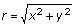the trigonometric functions are as follows.
Sin a = y/r
Cos a = x/r
Tan a = y/x
Csc a = r/ y
Sec a = r/x
Cot a =x/y
Trigonometric Equation
If the equation contains at least one trigonometric function that is true for some but not all values of the variables then that equation is called to be trigonometric equation.
Trigonometric Identity
If the equation contains a trigonometric function and is true for all the values of the variables then that equation is called to be trigonometric identity.
Trigonometric Identities includes 3 types, Quotient identities, Reciprocal identities, and Pythagorean identities.
Quotient Identities: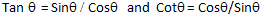Reciprocal Identities: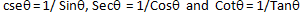Pythagorean Identities: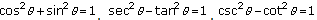Trigonometric Ratios(Same Definition) In ?ABC with right ?C,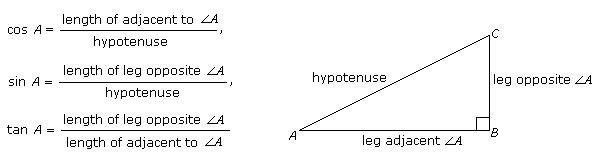Tangent Ratio
In a right angled triangle, the tangent of an angle is the ratio of the length of the side opposite to that angle to the length of the side adjacent to that angle.

### Solved Example on Trigonometry

#### Ques: Which of the following trigonometric ratios is the ratio between adjacent side and hypotenuse?

##### Choices:

A. cosine
B. tangent
C. sine
D. none of the above